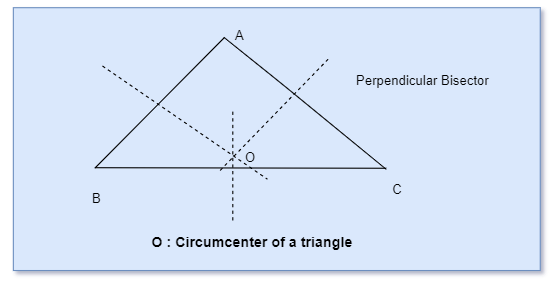# Circumcenter of a Triangle CalculatorNote
• Enter the values of Coordinates of the triangle
• Click on the calculate button.

Formula And concept used
1. Find the midpoints of the two sides
$x_m = \frac {x_1 + x_2}{2}$
$y_m = \frac {y_1 + y_2}{2}$
2. Find the perpendicular bisector lines to these side
3. The intersection of these two lines is the circumcenter

### Circumcenter of a Triangle Calculator

Example of Few questions where you can use this Centroid of a Triangle Calculator
1. Find the circumcenter of a Triangle.
i. A(2,3), B(4 ,5) , C(5,9)
ii. A(-1,3), B(5 ,8) , C(2,2)
iii. A(1,1), B(2 ,5) , C(1,7)
iv. A(2,3), B(5 ,4) , C(9,5)